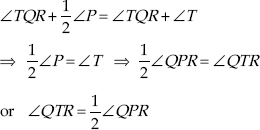# Lines and Angles (Mathematics) Class 9 - NCERT Questions

Q 1.

In the given figure, lines AB and CD intersect at O. If ∠AOC + ∠BOE = 70° and ∠BOD = 40°, find ∠BOE and reflex ∠COE.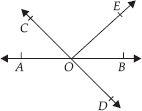SOLUTION:

Since AB is a straight line,
∴ ∠AOC + ∠COE + ∠EOB = 180°
or (∠AOC + ∠BOE) + ∠COE = 180°
or 70° + ∠COE = 180°
[∵ ∠AOC + ∠BOE = 70° (Given)]
or ∠COE = 180° – 70° = 110°
∴ Reflex ∠COE = 360° – 110° = 250°
Also, AB and CD intersect at O.
∴ ∠COA = ∠BOD [Vertically opposite angles]
But ∠BOD = 40° [Given]
∴ ∠COA = 40°
Also, ∠AOC + ∠BOE = 70°
∴ 40° + ∠BOE = 70°
or ∠BOE = 70° – 40° = 30°
Thus, ∠BOE = 30° and reflex ∠COE = 250°.

Q 2.

In the given figure, lines XY and MN intersect at O. If ∠POY = 90° and a : b = 2 : 3, find c.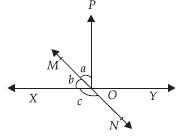SOLUTION:

Since XOY is a straight line.
∴ ∠b + ∠a + ∠POY = 180°
But ∠POY = 90° [Given]
∴ ∠b + ∠a = 180° – 90° = 90°
Also a : b = 2 : 3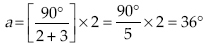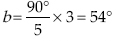Since XY and MN intersect at O,
∴ c = [a + ∠POY] [Vertically opposite angles]
or c = 36° + 90° = 126°
Thus, the required measure of c = 126°.

Q 3.

In the given figure, ∠PQR = ∠PRQ, then prove that ∠PQS = ∠PRT.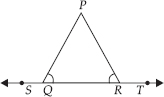SOLUTION:

ST is a straight line,
∴ ∠PQR + ∠PQS = 180° ...(1)
[Linear pair]
Similarly, ∠PRT + ∠PRQ = 180° ...(2)
[Linear Pair]
From (1) and (2), we have
∠PQS + ∠PQR = ∠PRT + ∠PRQ
But ∠PQR = ∠PRQ [Given]
∴ ∠PQS = ∠PRT

Q 4.

In the given figure, if x + y = w + z, then prove that AOB is a line.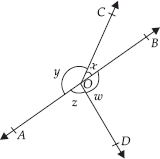SOLUTION:

Sum of all the angles at a point = 360°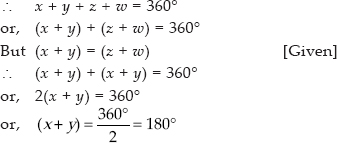∴ AOB is a straight line

Q 5.

In the given figure, POQ is a line. Ray OR is perpendicular to line PQ. OS is another ray lying between rays OP and OR. Prove that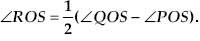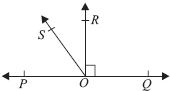SOLUTION:

POQ is a straight line.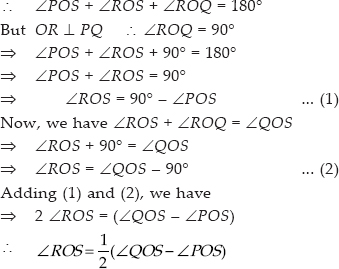Q 6.

It is given that ∠XYZ = 64° and XY is produced to point P. Draw a figure from the given information. If ray YQ bisects ∠ZYP, find ∠XYQ and reflex ∠QYP.

SOLUTION:

XYP is a straight line.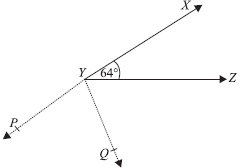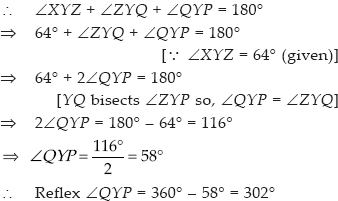Since ∠XYQ = ∠XYZ + ∠ZYQ ⇒ ∠XYQ = 64° + ∠QYP [∵ ∠XYZ = 64°(Given) and ∠ZYQ = ∠QYP] ⇒ ∠XYQ = 64° + 58° = 122° [∠QYP = 58°] Thus, ∠XYQ = 122° and reflex ∠QYP = 302°.

Q 7.

In the given figure, find the values of x and y and then show that AB ∥ CD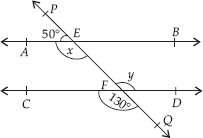SOLUTION:

In the figure, we have CD and PQ intersect at F.
∴ y = 130° ...(1)
[Vertically opposite angles]
Again, PQ is a straight line and EA stands on it.
∴ ∠AEP + ∠AEQ = 180° [Linear pair]
or 50° + x = 180°
⇒ x = 180° – 50° = 130° ... (2)
From (1) and (2), x = y
As they are pair of alternate interior angles.
∴ AB∥CD

Q 8.

In the given figure, if AB ∥ CD , CD ∥ EF and y : z = 3 : 7, find x.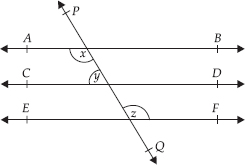SOLUTION:

AB∥ CD and EF ∥ CD [Given]
∴ AB ∥ EF and PQ is a transversal.
∴ x = z ... (1)
[Alternate interior angles]
Again, AB ∥ CD
⇒ x + y = 180° [Co-interior Angles]
⇒ z + y = 180° [By (1)]
But y : z = 3 : 7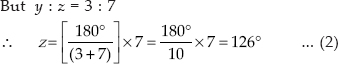From (1) and (2), we have
x = 126°.

Q 9.

In the given figure, if AB ∥ CD , EF ⊥ CD
and ∠GED = 126°, find ∠AGE, ∠GEF and ∠FGE.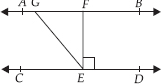SOLUTION:

AB CD and GE is a transversal.
∴ ∠AGE = ∠GED
[Alternate interior angles]
But ∠GED = 126° [Given]
∴ ∠AGE = 126°
Also, ∠GEF + ∠FED = ∠GED
or ∠GEF + 90° = 126°
[∵ EF ⊥ CD (given)]
∠GEF = 126° – 90° = 36°
Now, AB CD and GE is a transversal.
∴ ∠FGE + ∠GED = 180°
[Co-interior angles]
or ∠FGE + 126° = 180°
or ∠FGE = 180° – 126° = 54°
Thus, ∠AGE = 126°, ∠GEF = 36° and
∠FGE = 54°.

Q 10.

In the given figure, if PQ ST, ∠PQR = 110° and ∠RST = 130° , find ∠QRS.
[Hint: Draw a line parallel to ST through point R.]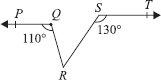SOLUTION:

Draw a line parallel to ST through R.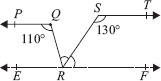Since PQ ∥ ST [Given]
and EF ∥ ST [Construction]
∴ PQ EF and QR is a transversal
⇒ ∠PQR = ∠QRF
[Alternate Interior Angles]
But ∠PQR = 110° [Given]
∴ ∠QRF = ∠QRS + ∠SRF = 110° ...(1)
Again ST EF and RS is a transversal
∴ ∠RST + ∠SRF = 180°
[Co-interior angles]
or 130° + ∠SRF = 180°
⇒ ∠SRF = 180° – 130° = 50°
Now, from (1), we have
∠QRS + 50° = 110°
⇒ ∠QRS = 110° – 50° = 60°
Thus, ∠QRS = 60°.

Q 11.

In the given figure, if AB CD, ∠APQ = 50° and ∠PRD = 127°, find x and y.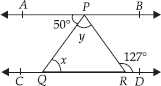SOLUTION:

We have AB CD and PQ is a transversal.
∴ ∠APQ = ∠PQR
[Alternate interior angles]
or 50° = x [∵ ∠APQ = 50° (Given)]
Again, AB CD and PR is a transversal.
∴ ∠APR = ∠PRD
[Alternate interior angles]
⇒ ∠APR = 127°
[∵ ∠PRD = 127° (given)]
⇒ ∠APQ + ∠QPR = 127°
⇒ 50° + y = 127°
[∵ ∠APQ = 50° (given)]
⇒ y = 127° – 50° = 77°
Thus, x = 50° and y = 77°.

Q 12.

In the given figure, PQ and RS are two mirrors placed parallel to each other. An incident ray AB strikes the mirror PQ at B, the reflected ray moves along the path BC and strikes the mirror RS
at C and again reflects back along CD. Prove that AB CD.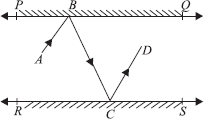SOLUTION:

Draw ray BL ⊥ PQ and CM ⊥ RS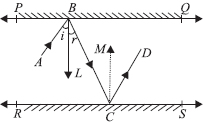∵ PQ ∥ RS ⇒ BL ∥ CM
[∵ BL ⊥ PQ and CM ⊥ RS]
Now, BL ∥ CM and BC is a transversal.
∴ ∠LBC = ∠MCB ...(1)
[Alternate interior angles]
Since, angle of incidence = Angle of reflection
∠ABL = ∠LBC and ∠MCB = ∠MCD
⇒ ∠ABL = ∠MCD ...(2)
∴ Adding (1) and (2), we have
∠LBC + ∠ABL = ∠MCB + ∠MCD
⇒ ∠ABC = ∠BCD
i.e., a pair of alternate interior angles are equal,
∴ AB ∥ CD.

Q 13.

In the adjoining figure, sides QP and RQ of ∆PQR are produced to points S and T respectively. If ∠SPR = 135° and ∠PQT = 110°, find ∠PRQ.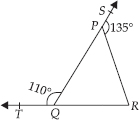SOLUTION:

We have, ∠TQP + ∠PQR = 180°
[Linear pair]
⇒ 110° + ∠PQR = 180°
⇒ ∠PQR = 180° – 110° = 70°
Since, the side QP of ∆PQR is produced to S.
⇒ ∠PQR + ∠PRQ = 135°
[Exterior angle property of a ∆]
⇒ 70° + ∠PRQ = 135° [∠PQR = 70°]
⇒ ∠PRQ = 135° – 70° ⇒ ∠PRQ = 65°

Q 14.

In the adjoining figure, ∠X = 62°, ∠XYZ = 54°. If YO and ZO are the bisectors of ∠XYZ and ∠XZY respectively of ∠XYZ, find ∠OZY and ∠YOZ.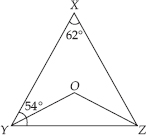SOLUTION:

In ∆XYZ, we have
∠XYZ + ∠YZX + ∠ZXY = 180°
[Angle sum property of a triangle]
But ∠XYZ = 54° and ∠ZXY = 62°
∴ 54° + ∠YZX + 62° = 180°
⇒ ∠YZX = 180° – 54° – 62° = 64°
YO and ZO are the bisectors of ∠XYZ and ∠XZY respectively,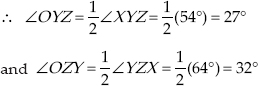Now, in ∆OYZ, we have
∠YOZ + ∠OYZ + ∠OZY = 180°
[Angle sum property of a triangle]
⇒ ∠YOZ + 27° + 32°= 180°
⇒ ∠YOZ = 180° – 27° – 32°= 121°
Thus, ∠OZY = 32° and ∠YOZ = 121°

Q 15.

In the given figure, if AB DE, ∠BAC = 35° and ∠CDE = 53°, find ∠DCE.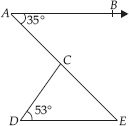SOLUTION:

AB DE and AE is a transversal.
So, ∠BAC = ∠AED [Alternate interior angles]
and ∠BAC = 35° [Given]
∴ ∠AED = 35°
Now, in ∆CDE, we have
∠CDE + ∠DEC + ∠DCE = 180°
[Angle sum property of a triangle]
∴ 53° + 35° + ∠DCE =180°
[∵ ∠DEC = ∠AED = 35° and ∠CDE = 53° (Given)]
⇒ ∠DCE = 180° – 53° – 35° = 92°
Thus, ∠DCE = 92°

Q 16.

In the adjoining figure, if lines PQ and RS intersect at point T, such that ∠PRT = 40°, ∠RPT = 95° and ∠TSQ = 75°, find ∠SQT.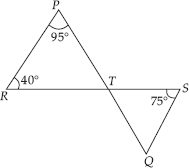SOLUTION:

In ∆PRT, we have
∠P + ∠R + ∠PTR = 180°
[Angle sum property of a triangle]
⇒ 95° + 40° + ∠PTR =180°
[∵ ∠P = 95°, ∠R = 40° (given)]
⇒ ∠PTR =180° – 95° – 40° = 45°
But PQ and RS intersect at T,
∴ ∠PTR = ∠QTS
[Vertically opposite angles]
∴ ∠QTS = 45° [∵ ∠PTR = 45°]
Now, in ∆TQS, we have
∠TSQ + ∠STQ + ∠SQT = 180°
[Angle sum property of a triangle]
∴ 75° + 45° + ∠SQT =180°
[∵ ∠TSQ = 75° and ∠STQ = 45°]
⇒ ∠SQT = 180° – 75° – 45° = 60°
Thus, ∠SQT = 60°

Q 17.

In the adjoining figure, if PQ ⊥ PS, PQ SR, ∠SQR = 28° and ∠QRT = 65°, then find the values of x and y.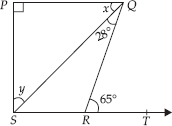SOLUTION:

In ∆QRS, the side SR is produced to T.
∴ ∠QRT = ∠RQS + ∠RSQ
[Exterior angle property of a triangle]
But ∠RQS = 28° and ∠QRT = 65°
So, 28° + ∠RSQ = 65°
⇒ ∠RSQ = 65° – 28° = 37°
Since, PQ SR and QS is a transversal.
∴ ∠PQS = ∠RSQ = 37°
[Alternate interior angles]
⇒ x = 37°
Again, PQ PS ⇒ ∠P = 90°
Now, in ∆PQS, we have
∠P + ∠PQS + ∠PSQ = 180°
[Angle sum property of a triangle]
⇒ 90° + 37° + y = 180°
⇒ y = 180° – 90° – 37° = 53°
Thus, x = 37° and y = 53°

Q 18.

In the adjoining figure, the side QR of ∆PQR is produced to a point S. If the bisectors of ∠PQR and ∠PRS meet at point That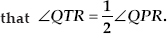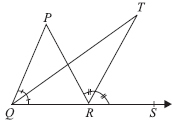SOLUTION:

In ∆PQR, side QR is produced to S, so by exterior angle property,
∠PRS = ∠P + ∠PQR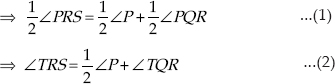[∵ QT and RT are bisectors of ∠PQR and ∠PRS respectively.]
Now, in ∆QRT, we have
∠TRS = ∠TQR + ∠T ...(3)
[Exterior angle property of a triangle]
From (2) and (3), we have